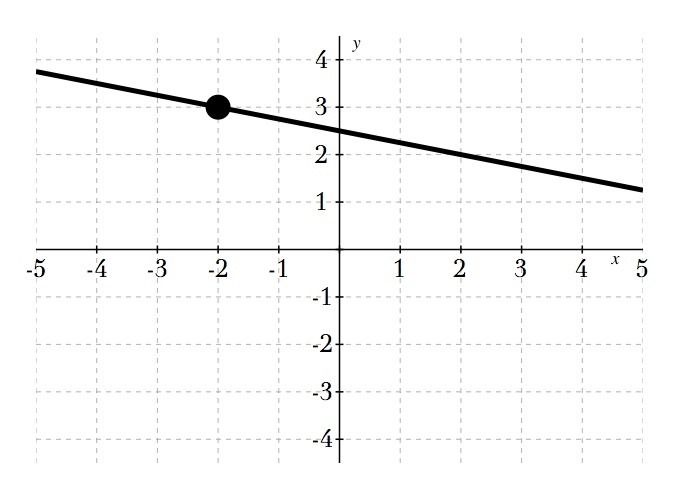Kindergarten
1st
2nd
3rd
4th
5th
6th
7th
8th
9th
10th
11th
12th
Higher Ed
Other
Subjects
ELA
Math
Science
Social Studies
Art
Computer Science
French
German
Music
Physical Education
Spanish
Other
Private Library
5.2 Point-Slope Form
starstarstarstarstarstarstarstarstarstar
by Jonathan Shaffer
| 22 Questions
Note from the author:
Assignment for using the point-slope formula and converting from point-slope to slope-intercept form.For the first six questions, type in the equation of the line in point-slope form. Do not use spaces.
1
2 pts
(1, -5); m=3
2
2 pts

3
2 pts

4
2 pts

5
2 pts

6
2 pts

Write the equation of the line in slope-intercept form.
7
3 pts

8
1 pt
Graph the equation above using the applet below
9
3 pts

10
3 pts

11
3 pts

12
3 pts

13
3 pts

14
1 pt
Graph the equation above using the graphing applet below.
For the next four questions, find the slope and use the point to write an equation of the line in point-slope form.15
3 pts
Write the equation of the line above.16
3 pts
What is the equation of the line above?17
3 pts
What is the equation of the line above?18
3 pts
What is the equation of the line above?
Write the equation of the line parallel to the given line in slope-intercept form.
19
3 pts

20
3 pts

21
3 pts

22
3 pts
Put the items in order below to take a point and a slope and turn it into a slope-intercept form equation.
1. Rearrange so the equation looks like "y=mx + b"
2. Get y alone but adding or subtracting the number on the left side
3. Input m and (x₁,y₂) into the point-slope equation. Covert any double negative signs to positives
4. Distribute the slope over the parentheses
Add to my formatives list